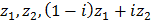# The triangle with vertices at the pointsis a) Right angled but not isosceles b) Isosceles but not right angled c) Right angled and isosceles d) Equilateral

## Question ID - 103328 :- The triangle with vertices at the pointsis a) Right angled but not isosceles b) Isosceles but not right angled c) Right angled and isosceles d) Equilateral

3537

(c)

Letbe the vertices of the triangle having affixesandrespectively. Then,Clearly,Hence,is isosceles right angled triangle

Next Question :
 On arranging water, sugar and oxygen in increasing order of attraction between their particles, which of the following will be the correct arrangement? (a) Water, Oxygen, Sugar (b) Oxygen, Sugar, Water (c) Sugar, Oxygen, Water (d) Oxygen, Water, Sugar